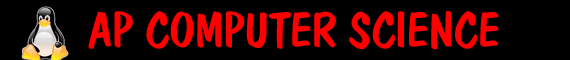Activity#1 - Primitive Data Exploration   In a class called PrimitiveData: What variable type and what number do you think this would equal 2+3/4? Program a method called testAdditionInt that would print out the results. What variable type and what number do you think this would equal 2+3.0/4? Program a method called testAdditionDouble that would print out the results. What variable type and what number do you think this would equal 10-(5+3.0)/4*2? Program a method called testOrderOfOperation that would print out the results. Program the method called divisibleByFive that will take in a number and if it is evenly divisible by 5 it will return true, otherwise it will return false. (if you have forgot methods) (if you have forgotten if statements Program the method printRows() that will print out the numbers from 1 to 100 with 10 numbers on each line(for loops) Program the method printRows(int begin, int end, int numEachRow) that will print out the numbers from begin to end with numEachRow on each line. Create a method called find least number of coins that will take as a parameter a number of cents (like 73) and it will print out "The least number of coins possible is 2 quarters, 2 dimes and 3 pennies". The header will be: public void leastNumOfCoins(int cents). ADV: Create a method called isSelfDivisor that given a 3 digit number, it will return true if every number in it goes evenly in the number, or false other wise. For example 122 is a self divisor (1 and 2 both evenly go in). Or 128 is a selfDivisor. If it has a 0 in it (ie 505), it is NOT a selfDivisor. Extra credit - heres the ap version (question 1) public void isSelfDivisor(int x) ADV: Create a method public void findMagicNumber() that satisfies this puzzle. Start with the code below: Create a new class in bluej and replace the code with the following: `````` /** * This class is to test primitive data structures */ public class PrimitiveDataExamples { /** * Use this to test simple addition of ints and print the result */ public void testAdditionInt() { //your code here System.out.println("here it is " + 3); } /** * Use this to test simple addition of ints and a double and print the result */ public void testAdditionDouble() { //your code here } /** * Use this to test order of operations */ public void testOrderOfOpp() { //your code here } /** * Use this to test if a number is evenly divisible by 5 * @param x the number to test if it is divisible * @return true if it is divisible by 5, false otherwise */ public boolean divisibleByFive(int x) { //your code here (currently it returns true) return true; } /** * Prints out numbers from 1-100 with 10 numbers on each line */ public void printRows() { //your code here } /** * Prints out numbers from begin-end with numEachRow numbers on each line * @param begin the number to begin at * @param end the number to end at * @param numEachRow the number of numbers on each row. */ public void printRows(int begin, int end, int numEachRow) { //your code here } /** * Prints out the least number of coins that it would take to make cents * @param cents the number of cents */ public void leastNumOfCoins(int cents) { //your code here } /** * given a 3 digit number, it will return true if every number in it goes evenly in the number, or false other wise. * For example 122 is a self divisor (1 and 2 both evenly go in). Or 128 is a selfDivisor. If it has a 0 in it (ie 505), it is NOT a selfDivisor. * @param x the number */ public boolean isSelfDivisor(int x) { //your code here (currently it returns true) return true; } /** * given any digit number, it will return true if every number in it goes evenly in the number, or false other wise. * For example 122 is a self divisor (1 and 2 both evenly go in). Or 128 is a selfDivisor. If it has a 0 in it (ie 505), it is NOT a selfDivisor. * @param x the number (which has any number of digits) */ public boolean APisSelfDivisor(int x) { //your code here (currently it returns true) return true; } //main method for Eclipse public static void main(String[] args) { PrimitiveDataExamples p=new PrimitiveDataExamples(); p.testAdditionInt(); p.testAdditionDouble(); p.testOrderOfOpp(); System.out.println("Divisible by 5 returns " + p.divisibleByFive(34)); p.printRows(); p.printRows(20,40,5); p.leastNumOfCoins(73); System.out.println("Is self divisor returns " + p.isSelfDivisor(122)); } } ``` ```